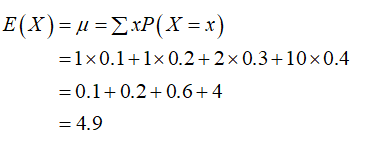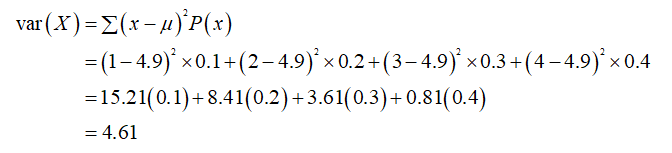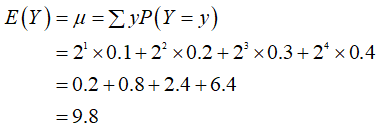# Let (S, P) be the sample space in which S = {1, 2, 3, 4} and P is given by P (1) = 0.1, P (2) = 0.2, P (3) = 0.3, and P (4) = 0.4.  Find the variance of the following random variables:a.     X is the random variable defined by X (1) = X (2) = 1, X (3) = 2, and X (4) = 10.b.     Y is the random variable defined by Y (k) = 2k (for k = 1, 2, 3, 4).c.     Z is the random variable defined by Z (k) = k2 (for k = 1, 2, 3, 4).

Question
1 views

Let (S, P) be the sample space in which S = {1, 2, 3, 4} and P is given by P (1) = 0.1, P (2) = 0.2, P (3) = 0.3, and P (4) = 0.4.  Find the variance of the following random variables:

a.     X is the random variable defined by X (1) = X (2) = 1, X (3) = 2, and X (4) = 10.

b.     Y is the random variable defined by Y (k) = 2k (for k = 1, 2, 3, 4).

c.     Z is the random variable defined by Z (k) = k2 (for k = 1, 2, 3, 4).

check_circle

Step 1

According to the given information, let (S,P) be the sample space in which S={1,2,3,4} and P is given by:

P(1) = 0.1

P(2) = 0.2

P(3) = 0.3

P(4) = 0.4

For part (a)

X is the random variable defined by X(1) = X(2) = 1, X(3) = 2 and X(4) = 10

It is required to calculate the variance of the following random variables.

The expected value is the sum of the product of each possibility x with its probability P(X=x):Step 2

Now, the variance is the expected value of the square deviation from the mean:Step 3

For part (b) Y is a random variable defined by Y(k) = 2k

It is required to calculate the variance.

The expected value is the sum of the product of each possibility x with its probability P(Y=y):...

### Want to see the full answer?

See Solution

#### Want to see this answer and more?

Solutions are written by subject experts who are available 24/7. Questions are typically answered within 1 hour.*

See Solution
*Response times may vary by subject and question.
Tagged in